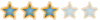# [討論]分享-統計選取範圍內之圖塊數量LISP##[討論]分享-統計選取範圍內之圖塊數量LISP

`　;　[BLOCK] COUNT THE NUMBER OF BLOCK(SELECTION SET:).　;　[圖塊] 統計選取範圍內圖塊數量。　;　支援軟體版本("AutoCAD")　;　設計者：Devin,Chou　;　版權所有，歡迎使用　;　Rev.1(2016/12/05-12/10)(defun C:bkc (/ lc_bm bm bm_lt                s_b sb_n s_bi bs_lt                bm_n bm_i bs_i )(setvar "cmdecho" 0 )(setq lc_bm 0 s_bi 0 bm_i 0 bs_i 0 bm_lt '() bs_lt '() )(while (= lc_bm 0 )(if (= bm_lt nil )    (setq bm_lt    (cons    (cdr    (assoc 2    (tblnext "BLOCK" T ))) bm_lt ))    (progn    (if (= (setq bm (tblnext "BLOCK" )) nil )    (setq lc_bm 1 )    (setq bm_lt    (cons    (cdr    (assoc 2 bm )) bm_lt )))))) ; if.end,progn.end,if.end,while-lc_bm.end(princ "\n請選取圖塊:" )(setq s_b    (ssget '((0 . "INSERT" ))))(repeat (sslength s_b )(setq sb_n    (cdr    (assoc 2    (entget    (ssname s_b s_bi )))))(setq bs_lt    (cons sb_n bs_lt ))(setq s_bi    (1+ s_bi ))) ; repeat.end(while (< bm_i (length bm_lt ))(setq bm_n    (nth bm_i bm_lt ))(foreach n bs_lt    (if (= n bm_n )    (setq bs_i    (1+ bs_i )))) ; if.end,foreach.end(if (/= bs_i 0 )    (progn    (princ    (strcat "\n" bm_n "：" (itoa bs_i ) "個" ))    (setq bs_i 0 ))) ; progn.end,if.end(setq bm_i    (1+ bm_i ))) ; while-bm_lt.end(textscr)(prin1)) ; bkc.end(princ "\nC:bkc")(prin1)`devinchou##回復： [討論]分享-統計選取範圍內之圖塊數量LISP

devinchou 寫到:看了版上的前輩分享了圖塊數量統計的LISP,Tiger&蘋果爸##回復： [討論]分享-統計選取範圍內之圖塊數量LISPmayaguy##回復： [討論]分享-統計選取範圍內之圖塊數量LISP謝謝分享。Pauline##回復： [討論]分享-統計選取範圍內之圖塊數量LISPlin6512_07